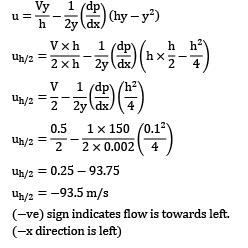Courses

# Test: Viscous Flow of Incompressible Fluids, Flow Losses Level - 2

## 20 Questions MCQ Test Engineering Mechanics | Test: Viscous Flow of Incompressible Fluids, Flow Losses Level - 2

Description
This mock test of Test: Viscous Flow of Incompressible Fluids, Flow Losses Level - 2 for Civil Engineering (CE) helps you for every Civil Engineering (CE) entrance exam. This contains 20 Multiple Choice Questions for Civil Engineering (CE) Test: Viscous Flow of Incompressible Fluids, Flow Losses Level - 2 (mcq) to study with solutions a complete question bank. The solved questions answers in this Test: Viscous Flow of Incompressible Fluids, Flow Losses Level - 2 quiz give you a good mix of easy questions and tough questions. Civil Engineering (CE) students definitely take this Test: Viscous Flow of Incompressible Fluids, Flow Losses Level - 2 exercise for a better result in the exam. You can find other Test: Viscous Flow of Incompressible Fluids, Flow Losses Level - 2 extra questions, long questions & short questions for Civil Engineering (CE) on EduRev as well by searching above.
QUESTION: 1

### A piping system consists of three pipes arranged in series; the lengths of the pipes are 1200 m, 750 m and 600 m and diameters 750 mm, 600 mm and 450 mm respectively.Transform the system to an equivalent 450 mm diameter pipe

Solution: l1 = 1200 m, l2 = 750 m, l3 = 600 m

d1 = 750 mm d2 = 600 mm d3 = 450 mm

de = 450 mm

For pipes in series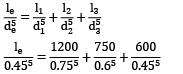le = 871 m

QUESTION: 2

### A fluid jet is discharging from a 100 mm nozzle and the vena contracta formed has a diameter of 90 mm. If the coefficient of velocity is 0.95, then the coefficient of discharge for the nozzle is

Solution: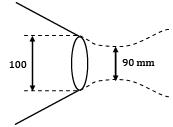CV = Vcontracta actual / Vcontracta Theory = 0.95

Q actual = Vcontracta actual × Acontracta

= VC,Theory × CV × AC / Anozzle × Anozzle

Qactual = VC,Theory × Anozzle × CV × AC / Anozzle

Now CV × AC / AN = Cd

where Cd = Coefficient of discharge

So Cd = 0.95 × 902 / 1002

Cd = 0.7695

QUESTION: 3

### Oil at 20°C (ρ = 888 kg⁄m3 and μ = 0.800 kg ⁄ ms) is flowing steadily through a 5-cm-diameter 40-m-long pipe as shown in the below figure. The pressure at the pipe inlet and outlet are measured to be 745 and 97 kPa, respectively. Determine the flow rate of oil through the pipe (in m3/s) assuming the pipe is inclined 15° upward.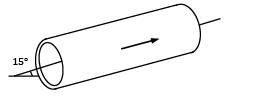Solution: In this question the total pressure drop will be the addition of static pressure drop and the piezometric pressure drop.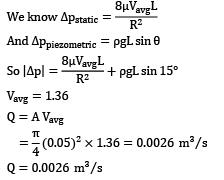QUESTION: 4

Consider the diagram below.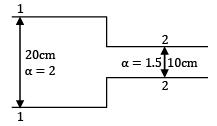ρ = 1000 kg/m3 = constant

If pressure at 1 = 150 kPa and pressure at 2 = 100 kPa, the loss coefficient = 0.5 and discharge = 0.01 m3 /s. Calculate the major head loss, correct upto 2 decimal places (in m)

Solution: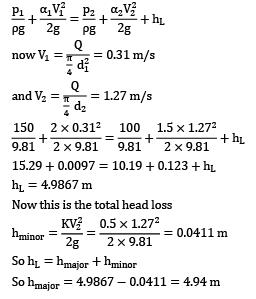QUESTION: 5

Where does the point of maximum instability exist for laminar flow through a pipe of radius R?

Solution: The velocity gradient for laminar flow through a pipeline is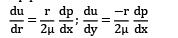Where the radial distance r from the pipe axis is r = R − y

The dimensionless parameter x is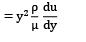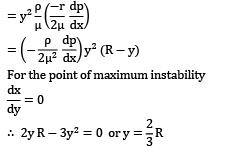Hence the point of maximum instability exists at a distance two-third of pipe radius from the wall.

QUESTION: 6

The value of kinetic energy correction factor for laminar flow through a circular pipe is approximately equal to

Solution: Kinetic energy correction factor,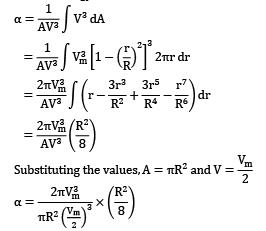⇒ α = 2

QUESTION: 7

Water is discharged from a tank maintained at a constant head of 5 m above the exit of a straight pipe 100 m long and 15 cm in diameter. If the friction coefficient for the pipe is 0.01, the rate of flow will be nearly

Solution: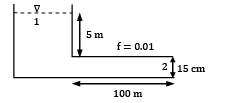Applying Bernoulli’s equation at 1 and 2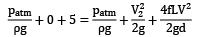Note: f = friction coefficient (CF) not Darcy friction factor.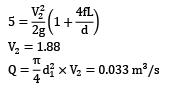QUESTION: 8

An oil of viscosity 8 poise flows between two parallel fixed plates, which are kept at a distance of 30 mm apart. If the drop of pressure for a length of 1 m is 0.3 × 104 N⁄m2 and width of the plates is 500 mm, the rate of oil flow between the plates will be

Solution: μ = 0.8; h = 30 mm; L = 1 m Δp = −0.3 × 104 N⁄m2 w = 500 mm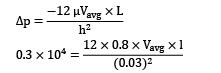Vavg = 0.281 m/s

Q = h × w × Vavg

= 0.03 × 0.5 × 0.281

= 0.00421m

= 4.21 × 10−3m

QUESTION: 9

With reference to section ① and ② consider the following statements, neglecting all losses.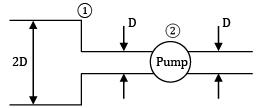Solution: HGL joins the piezometric heads at all points in flow while TEL joins the total energy head at all points in flow.

∴ TEL = HGL + V2 / 2g at a particular point

!t section ① due to sudden contraction, velocity head increases but the total energy remains constant, (as all loses are neglected) therefore HGL will drop but TEL will remain constant. !t section ② external energy is supplied to the fluid with the help of a pump, due to which both HGL and TEL will rise.

Note: Since the diameter of the pipe is the same on either side of the pump, velocity head remains constant on either side of the pump.

QUESTION: 10

Considering all the major and minor losses in the pipe network, the total head loss is (Assume Q and Darcy friction factor, f is a constant) __________ m.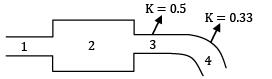1: Length = 200 cm, diameter = 10 cm

2: Length = 2.5 m, diameter = 20 cm

3: Length = 1 m, diameter = 15 cm Loss coefficient = 0.5

4: Mean length = 500 cm, diameter = 15 cm Loss coefficient = 0.33

Q = 0.1 m3 /s, f = 0.05. Assume flow is laminar everywhere.

Solution: Total head loss = Σ Hmajor + Σ Hminor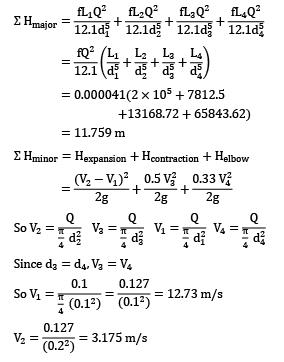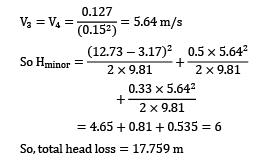QUESTION: 11

Two reservoirs connected by two pipelines in parallel of the same diameter D and length l. It is proposed to replace the two pipelines by a single pipeline of the same length l, without affecting the total discharge and loss of head due to friction. The diameter of the equivalent pipe De in terms of the diameter of the existing pipeline, De / D is

Solution: Given that hf is same for both cases QTotal = same for both cases Length of pipe is same for both cases For parallel pipes we know hf1 = hf2 So,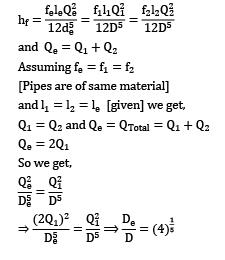QUESTION: 12

Consider fully developed laminar flow in a circular pipe of a fixed length

1. The friction factor is inversely proportional to Reynolds number

2. The pressure drop in the pipe is proportional to the average velocity of the flow in the pipe

3. The friction factor is higher for a rough pipe as compared to a smooth pipe

4. The pressure drop in the pipe is proportional to the square of average of flow in the pipe

What of the above statements are correct?

Solution: 1 is true, 2 is true, 3 is false as friction factor only depends on Reynolds number of the pipe and has nothing to do with roughness of pipe. 4 is false as pressure drop is directly proportional U and U × !rea = Q. So, if area is constant then U is proportional to Q and hence pressure drop is also proportional to Q.

*Answer can only contain numeric values
QUESTION: 13

The space between two plates (20cm*20cm*1cm), 1 cm apart, is filled with a liquid of viscosity 1 Poise. The upper plate is dragged to the right with a force of 5N keeping the lower plate stationary.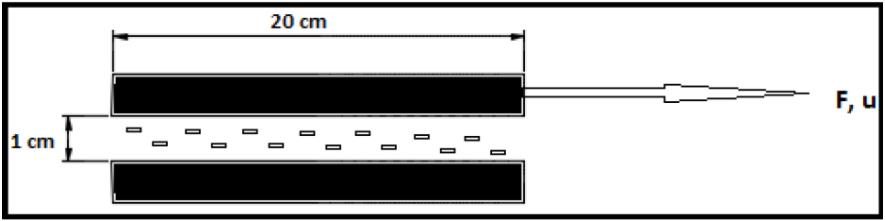What will be the velocity in m/s of flow at a point 0.5 cm below the lower surface of the upper plate if linear velocity profile is assumed for the flow?

Solution: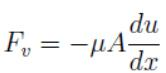where Fν = viscous force, A = area, du ⁄ dx = velocity gradient, μ = coefficient of viscosity. If linear velocity profile is assumed, du⁄dx = U/x, where U = velocity of the upper plate and x = distance between the two plates. Now, the viscous force Fv = -F= -5N. Substituting all the values in the equation, U becomes 12.5 m/s.

QUESTION: 14

Consider the case of minor head loss due to sudden contraction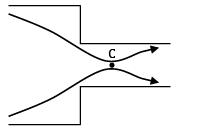(here C is the point of vena contracta)

Which of the following is correct?

Solution: The minor head loss due to sudden contraction occurs downstream of C because of the presence of adverse pressure gradient (dp /dx > 0) which causes flow separation.

QUESTION: 15

A 0.20 m diameter pipe 20 km long transports oil at a flow rate of 0.01 m3⁄s. Calculate power required to maintain flow if dynamic viscosity and density of oil is 0.08 Pas and 900 kg⁄m3 respectively.

Solution: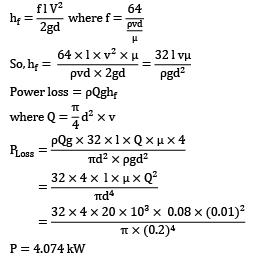QUESTION: 16

Consider flow of oil and water through a channel; the boundary conditions at the interface are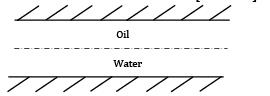Solution: Since the interface will have a defined velocity (Vi). The velocity of a particle just below the interface (in water) has to be the same as the velocity of the particle just above the interface (in oil), otherwise the interface will deform due to uneven velocity. Assuming the flow is fully developed. The shear stress (τ) just above and below the interface must be the same, or there will be a net force on the interface which will accelerate the interface. Consider an element at the interface.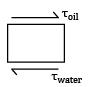For uniform velocity (τoil = τwater ) at interface Since τ is equal at interface in both the liquids, shear stress is continuous.

Since the interface has a defined velocity, velocity will be continuous.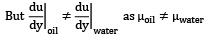QUESTION: 17

Consider the image below.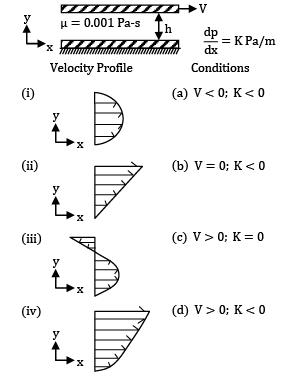Codes: (i) (ii) (iii) (iv)

Solution: The general velocity profile for Couette flow is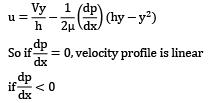then V = 0 (Parabolic)

V < 0="" gives="" (iii)="" and="" v="" /> 0 gives (iv)

QUESTION: 18

Assertion (A): The power transmitted through a pipe is maximum when the loss of head due to friction is equal to one-third of total head at the inlet.

Reason (R): Velocity is maximum when the friction loss is one-third of the total head at the inlet.

Solution: Maximum velocity can only be obtained when head loss is zero and the flow is ideal (no head loss). In viscous flow through pipes, head loss = initial head/3 is a condition for maximum power and not for maximum velocity. Assertion is true, Reason is false.

QUESTION: 19

Which one of the following is incorrect for the developing region of the pipe flow?

Solution: Assuming constant discharge and incompressible flow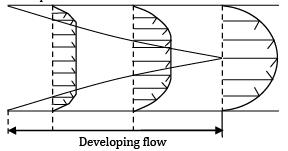Clearly, the velocity profile is more parabolic downstream. The velocity near the centre is increasing, so the fluid has convective acceleration.

By continuity equation Q entry = Q developing region = Q developed region So ! × U = A × Uavg,developing = A × Uavg Since A is constant, as diameter of pipe is constant.

U∞ = Uavg,developing = Uavg = Umax / 2

So the average velocity stays constant, and velocity at the centre line is the maximum velocity (Umax ) which is always greater than free stream velocity (U).

QUESTION: 20

For viscous, incompressible flow between two flat plates as shown, the velocity at y = h / 2 is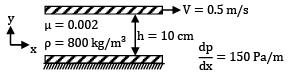Solution: For Couette flow, the general velocity profile is given as